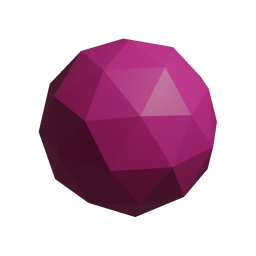## Limits

← Back to the main page

Another topic of basic calculus are limits, both one- and two-sided.

## Two-sided limits

The first method to find limits is method Limit(Variable, Entity). Example:
Entity expr = "(a x3 + b x2 + c) / (h x3 - b x - 1)";
Console.WriteLine(expr.Limit("x", "+oo"));
Output:
a / h
That is, as the first argument a variable should be passed, and its destination - as the second argument.

Symbols +oo and -oo are parsed as real positive and real negative infinities respectively.

Not only it works with rational functions, here are a few examples of more advanced cases. Example:
// First remarkable
Entity expr = "sin(a x) / x";
Console.WriteLine(expr.Limit("x", "0"));// First remarkable
Entity expr2 = "sin(a x) / tan(b x)";
Console.WriteLine(expr2.Limit("x", "0"));// Second remarkable
Entity expr3 = "(1 + a / x)^(d x)";
Console.WriteLine(expr3.Limit("x", "+oo"));// l'Hopital's rule
Entity expr4 = "(tan(t) - sin(t)) / t3";
Console.WriteLine(expr4.Limit("t", "0"));
Output:
a
a / b
e ^ (a * d)
1/2

If a limit cannot be found, a note of Entity.Limitf is returned:
Entity expr = "(sin(t) - tan(t)) / t";
Console.WriteLine(expr.Limit("t", "+oo"));
Output:
limit((sin(t) - tan(t)) / t, t, +oo)

Extension: string.Limit(Variable, Entity)

## One-sided limits

The method is Limit(Variable, Entity, AngouriMath.Core.ApproachFrom), where ApproachFrom is a enum of three possible values: BothSides, Left, Right. If BothSided is passed, it will work same as Limit(Variable, Entity). Otherwise, we shall consider now. Simple example:
Entity expr = "(a x2 + b) / (c x2 + d)";
Console.WriteLine(expr.Limit("x", "3", AngouriMath.Core.ApproachFrom.Left));
Output:
(a * 9 + b) / (c * 9 + d)

Another example:
Entity expr = "(a x2 + b) / (c x2 + d)";
Console.WriteLine(expr.Limit("x", "0", AngouriMath.Core.ApproachFrom.Left));
Output:
b / d

Extension: string.Limit(Variable, Entity, ApproachFrom).

As for now, 17.03.2021, AM does not support advanced algorithms of finding limits. Make sure to stay on the most recent version to track updates.

2019-2021 Angouri · Project's repo · Site's repo · Octicons